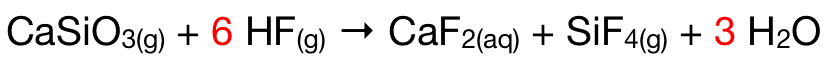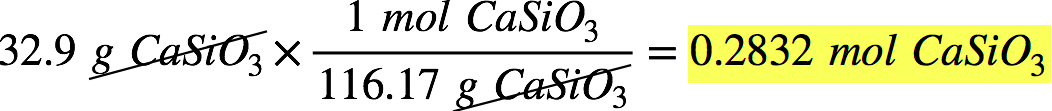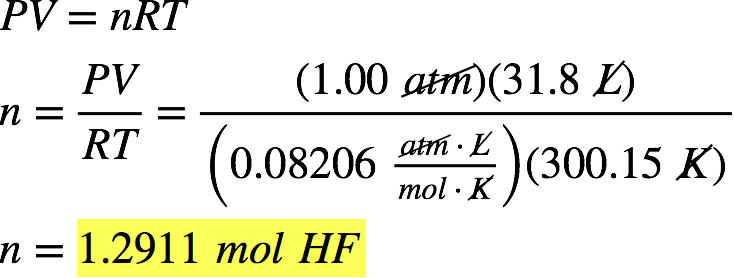# Problem: Consider the unbalanced chemical equation below:CaSiO3(s) + HF(g) → CaF2(aq) + SiF4(g) + H2O(l)Suppose a 32.9-g sample of CaSiO3 is reacted with 31.8 L of HF at 27.0°C and 1.00 atm. Assuming the reaction goes to completion, calculate the mass of the SiF4 and H2O produced in the reaction.

###### FREE Expert Solution

We first need to balance the given chemical equation. We add a coefficient of 6 to HF to balance the number of F on both sides of the equation. We also add a coefficient of 3 to H2O to balance the number of H and O. The balanced chemical equation is:We then need to determine the moles of CaSiO3 and HF given. The molar mass of CaSiO3 is 40.08 g/mol Ca + 28.09 g/mol Si + 3(16.00 g/mol O) = 116.17 g/mol. The moles of CaSiO3 given is:We can calculate the moles of HF using the ideal gas law. We're given P = 1.00 atm, V = 31.8 L, and T = 27.0°C = 300.15 K.Since we're given the amounts of both reactants, we need to determine which between CaSiO3 and HF is the limiting reactant. The limiting reactant forms the least amount of products amount reactants and actually determines the amount of products formed.

The molar mass of SiF4 is 28.09 g/mol Si + 4(19.00 g/mol F) = 104.09 g/mol and the molar mass of H2O is 2(1.01 g/mol H) + 16.00 g/mol O = 18.02 g/mol.

100% (469 ratings)###### Problem Details

Consider the unbalanced chemical equation below:

CaSiO3(s) + HF(g) → CaF2(aq) + SiF4(g) + H2O(l)

Suppose a 32.9-g sample of CaSiO3 is reacted with 31.8 L of HF at 27.0°C and 1.00 atm. Assuming the reaction goes to completion, calculate the mass of the SiF4 and H2O produced in the reaction.